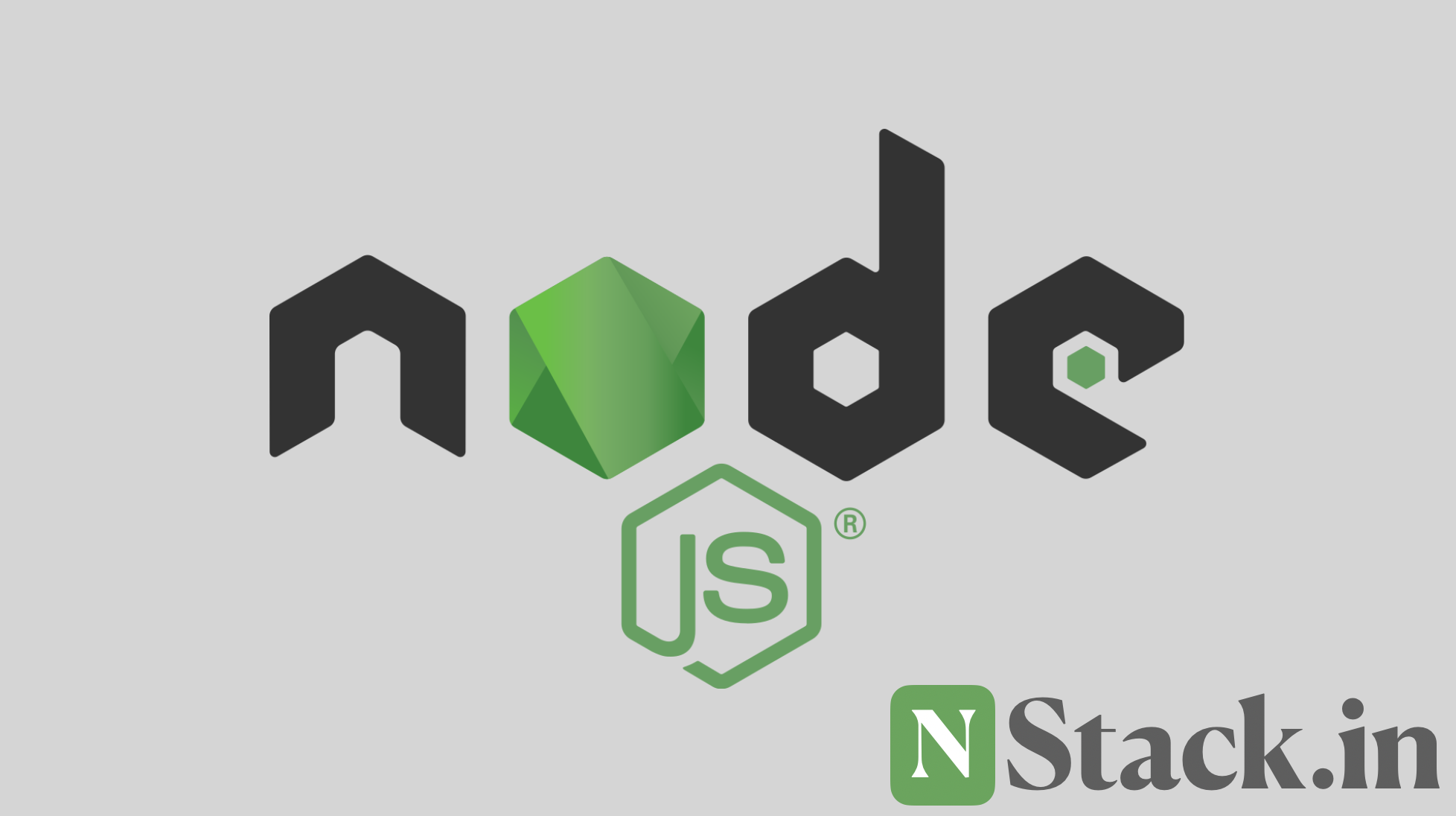# Functions in Node.js #

Function is one of the important part of funcitonal programming which makes our life easier.

The classic way of defining the function is here. This is better and I will more because it allow the function to be hoisted.

``````function add(num, num2) {
let result = num + num2;
return result;
}
``````

Here the function is hoisted, means we can call the function before decanting

There is more way of doing this and it’s interesting. You can store the function in any variable.

``````var sum = function (num1, num2) {
let result = num + num2;
return result;
};
``````

Here the function is not hoisted, means we can not call the function before decanting